# RS Aggarwal Solutions for Class 8 Maths Chapter 22 - Introduction to Coordinate Geometry Exercise 22A

Students can download the RS Aggarwal Solutions for the Exercise 22A of Class 8 Maths Chapter 22, Introduction to Coordinate Geometry from the link given below. Rehearsing as many times as possible helps in building time management skills and also boosts the confidence level to achieve high marks. To accomplish this, BYJU’S expert has solved the questions in different ways. This exercise includes the basic concept of the cartesian plane. By practising the RS Aggarwal Solutions for Class 8, students will be able to grasp the concepts entirely. Hence, students whose aim is to score high in Maths examinations are advised to go through RS Aggarwal Solutions for Class 8.

## Download PDF of RS Aggarwal Solutions for Class 8 Maths Chapter 22 – Introduction to Coordinate Geometry – Exercise 22A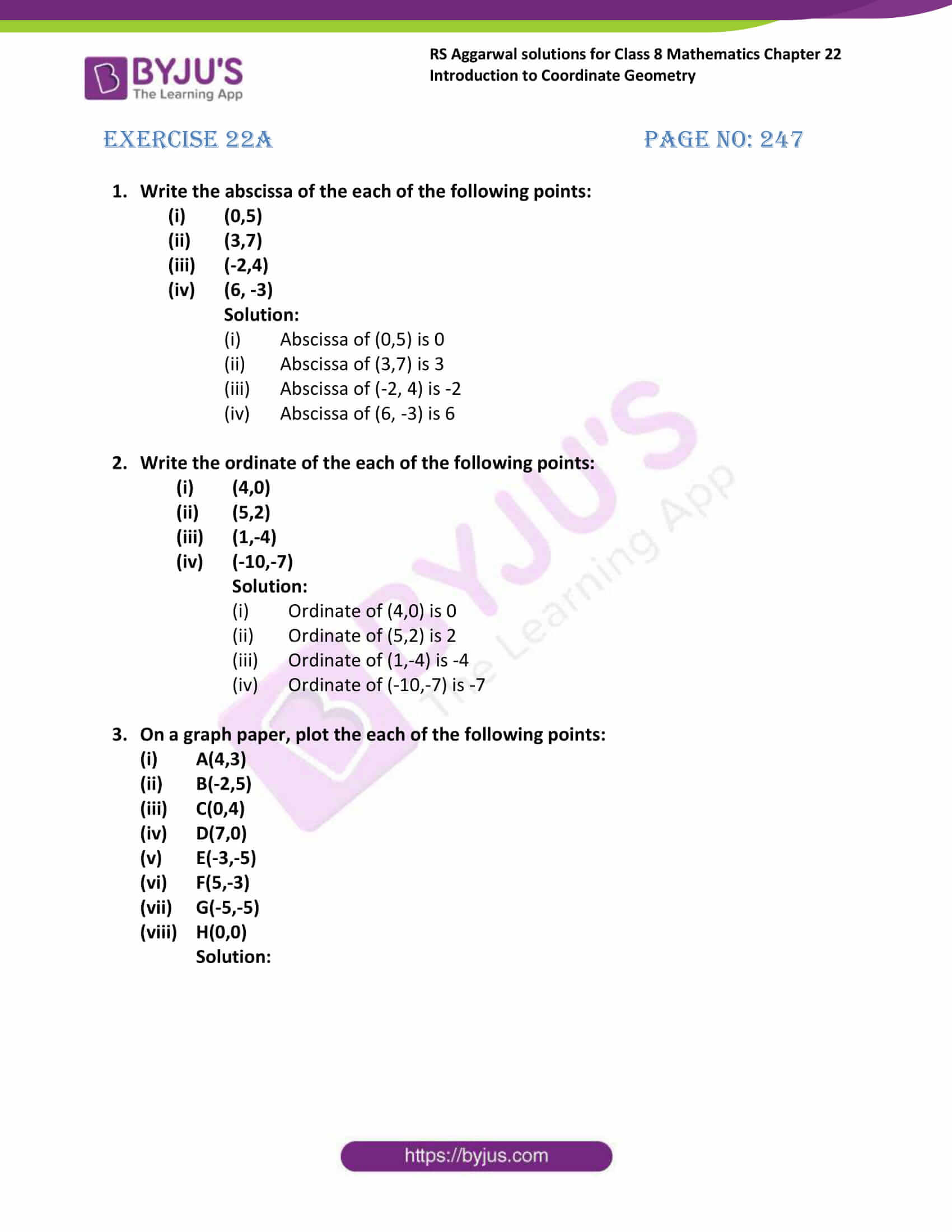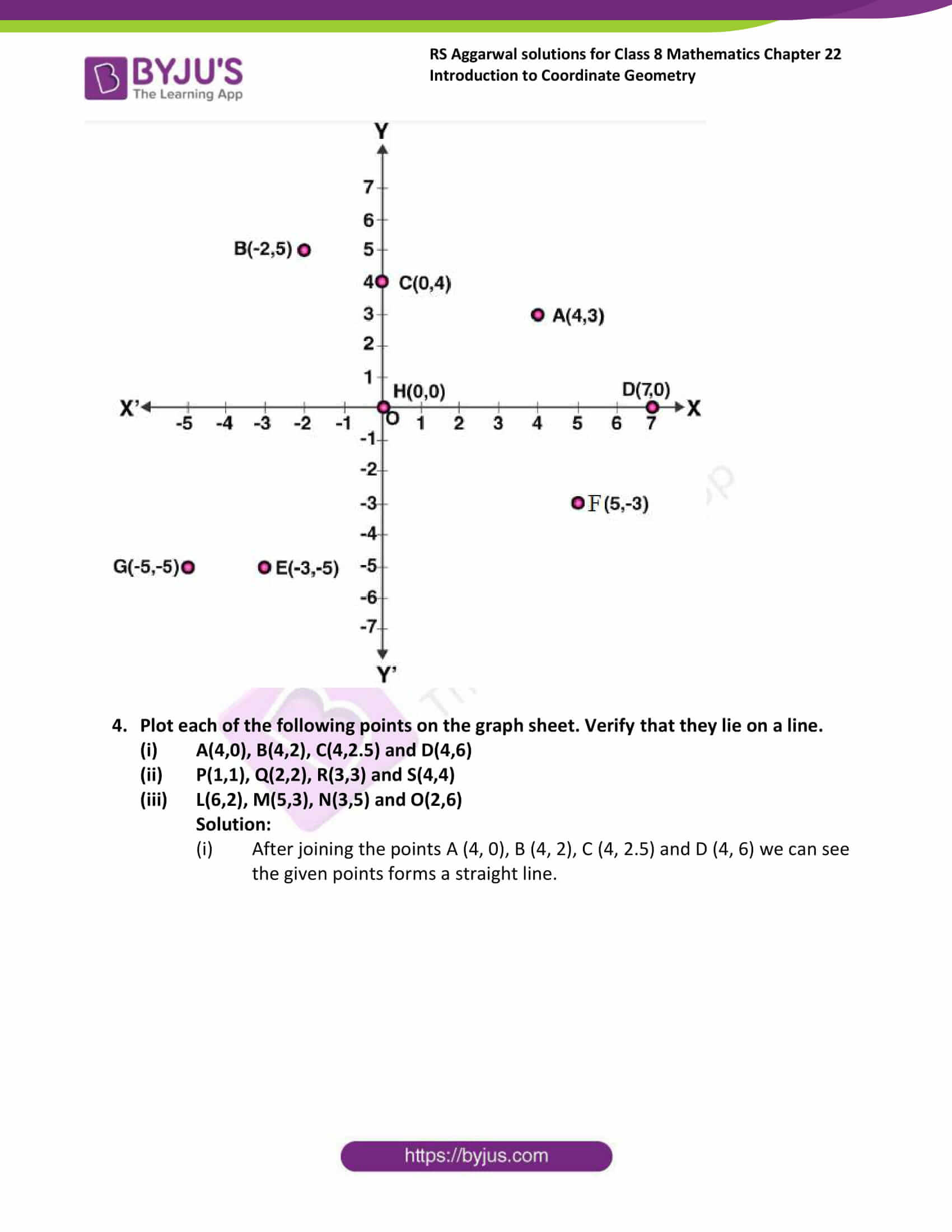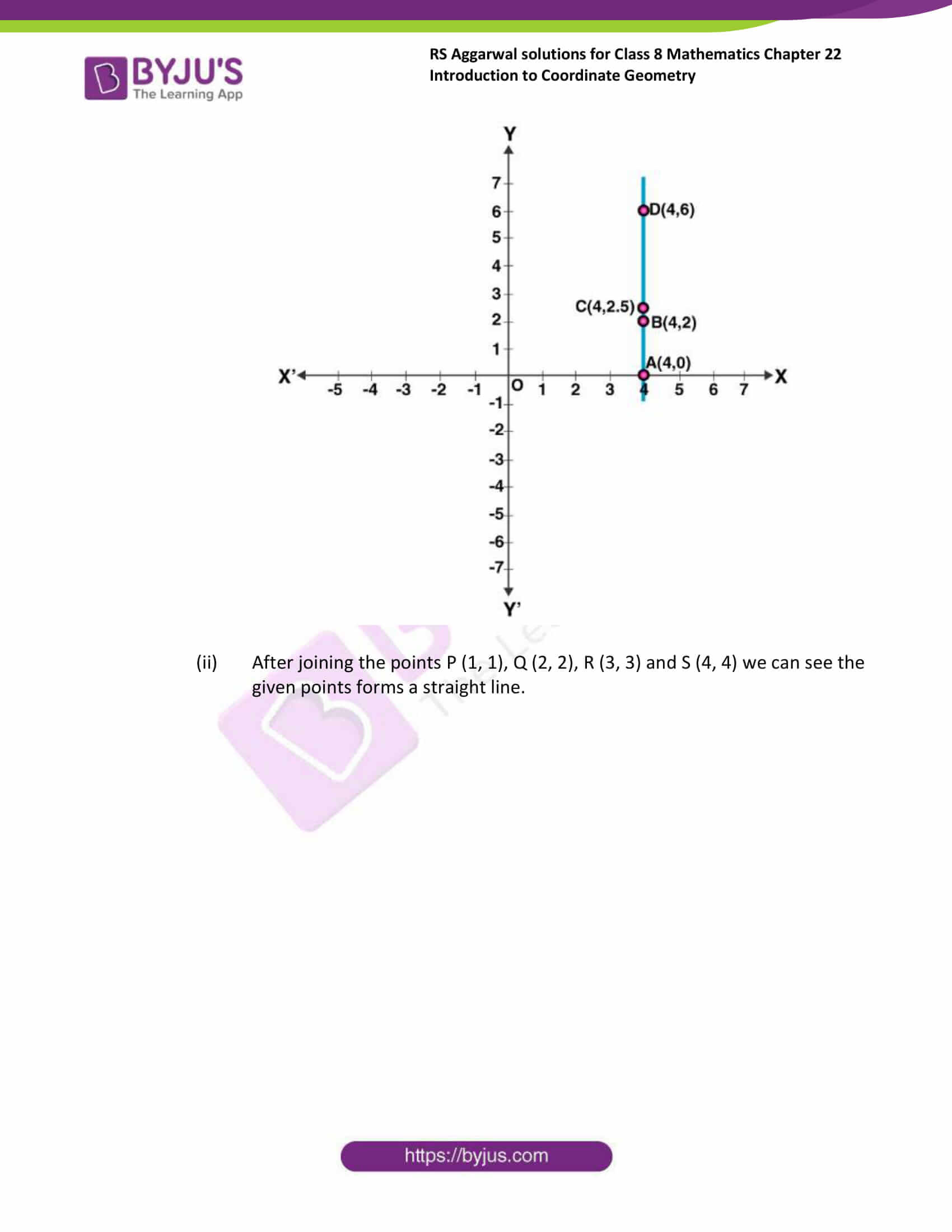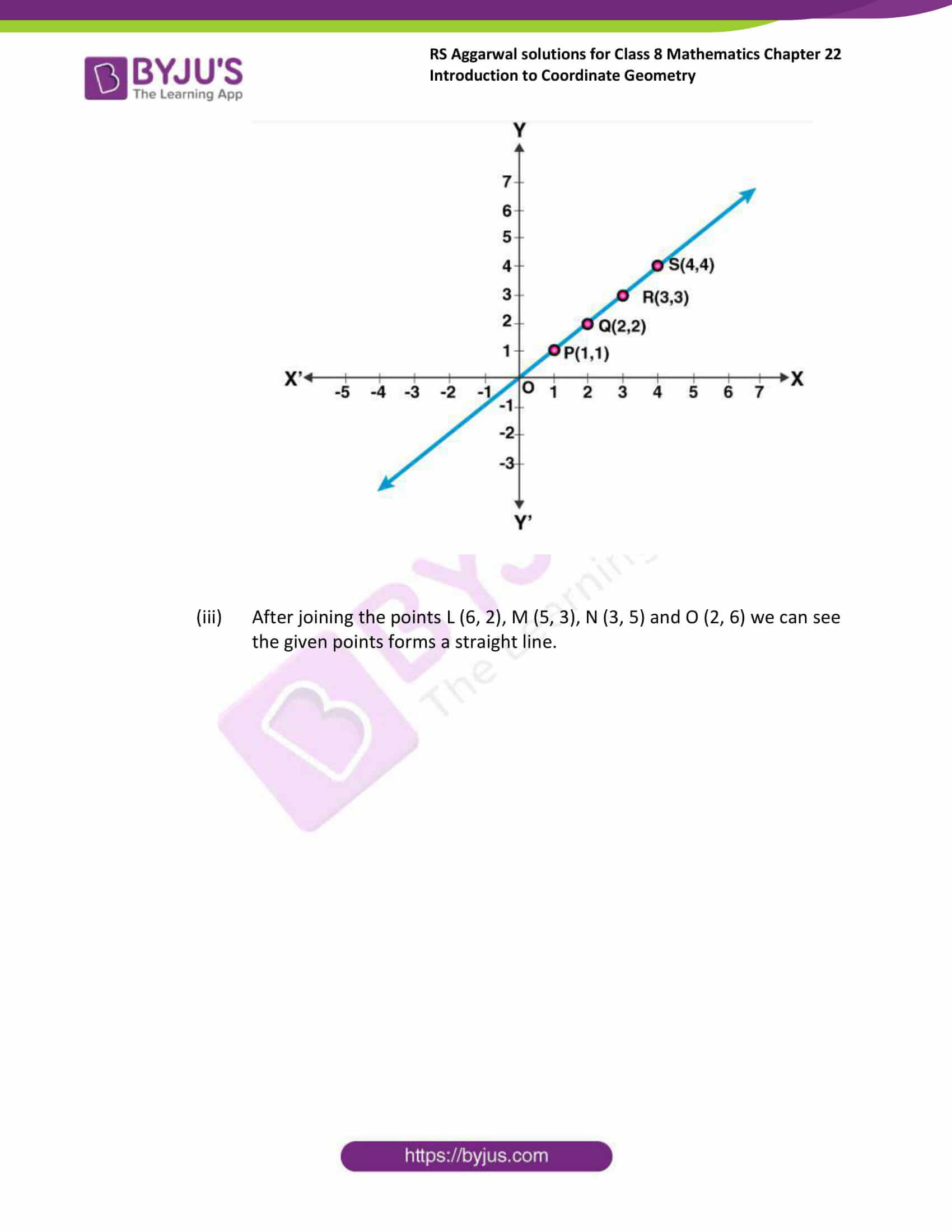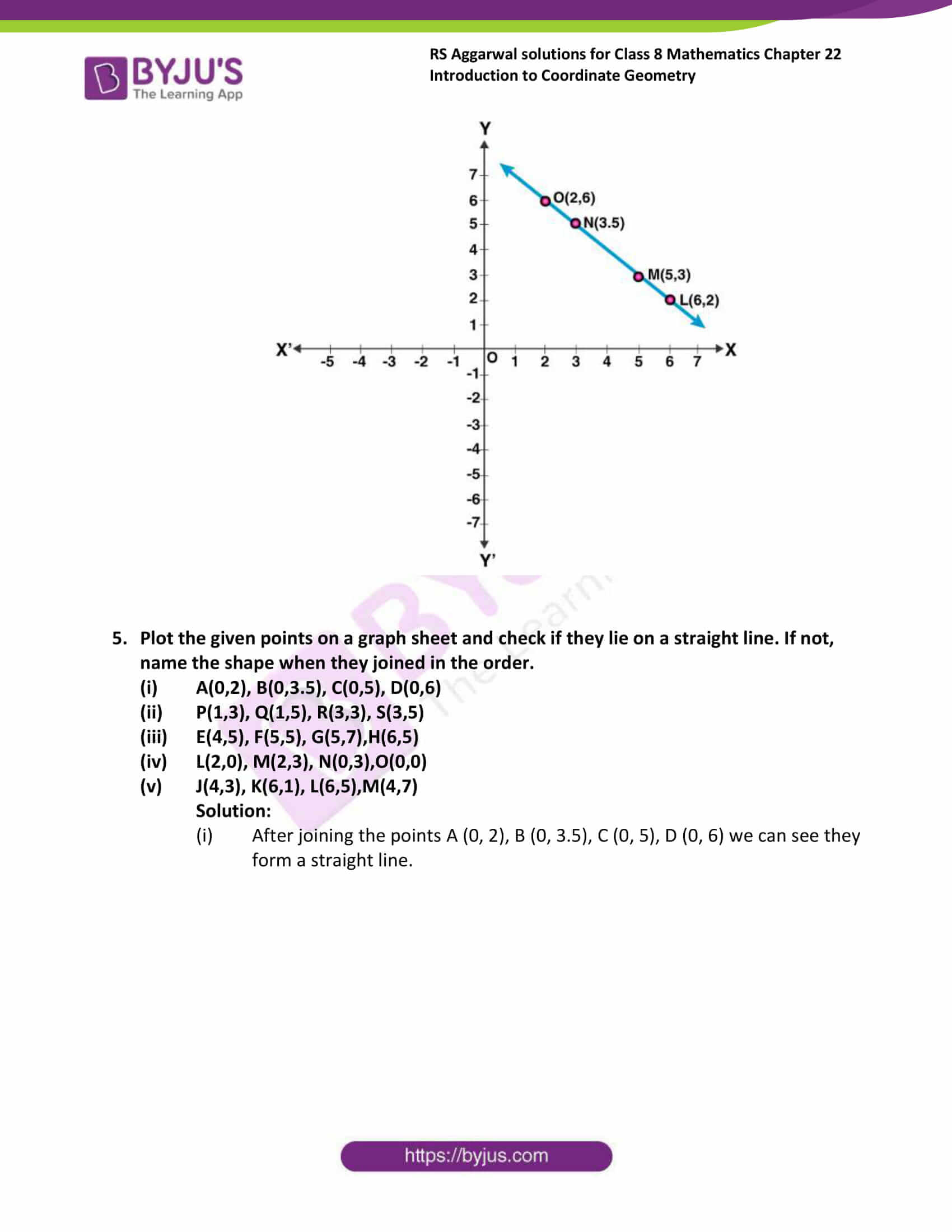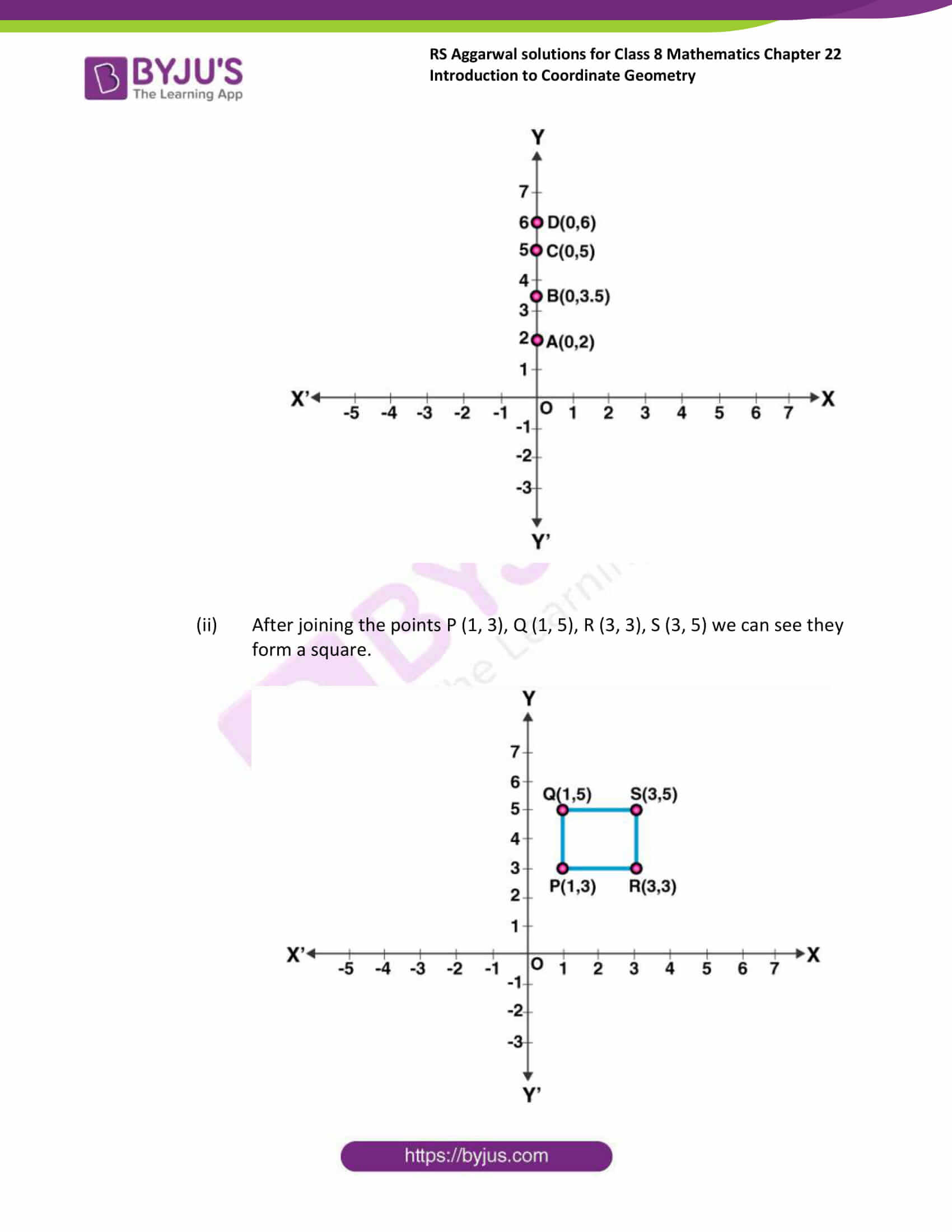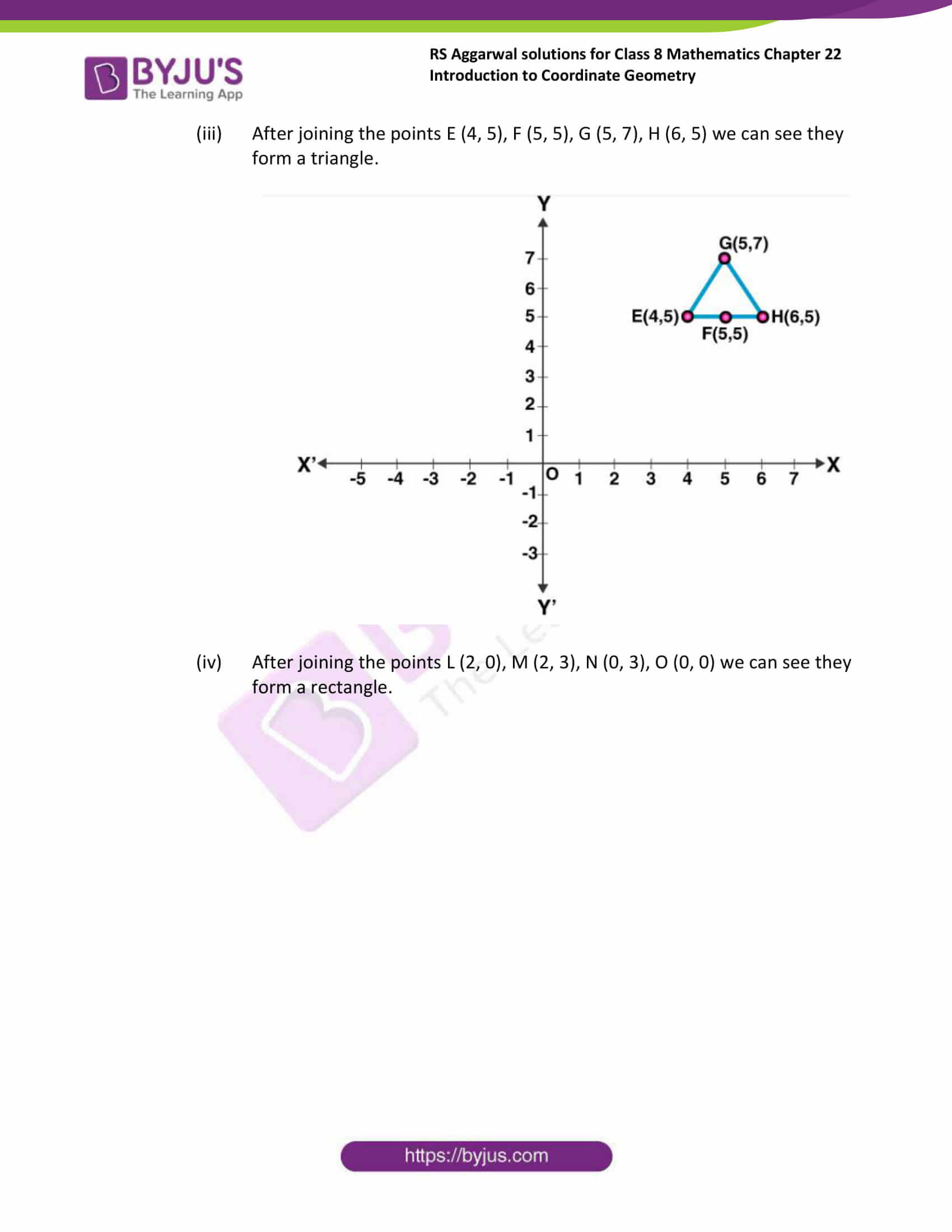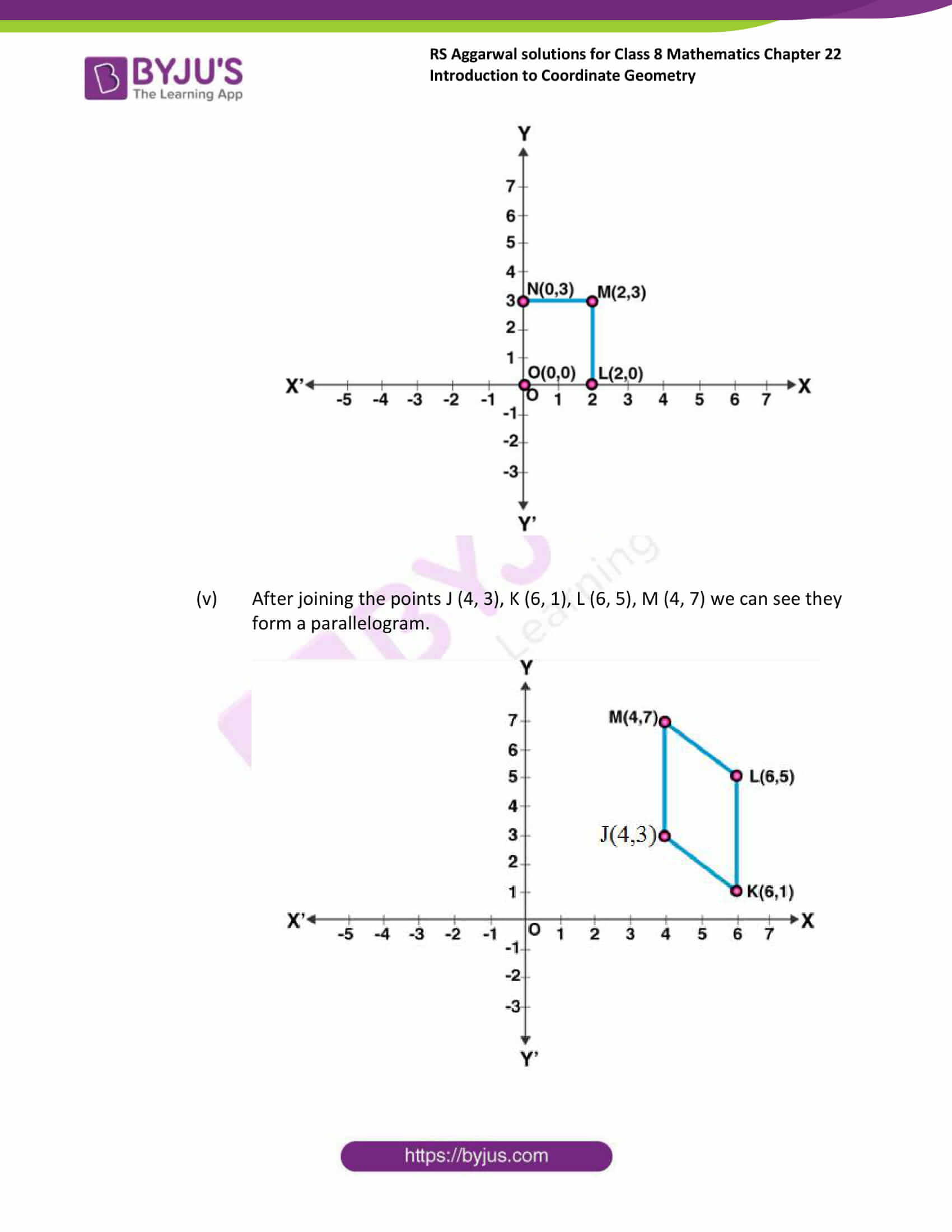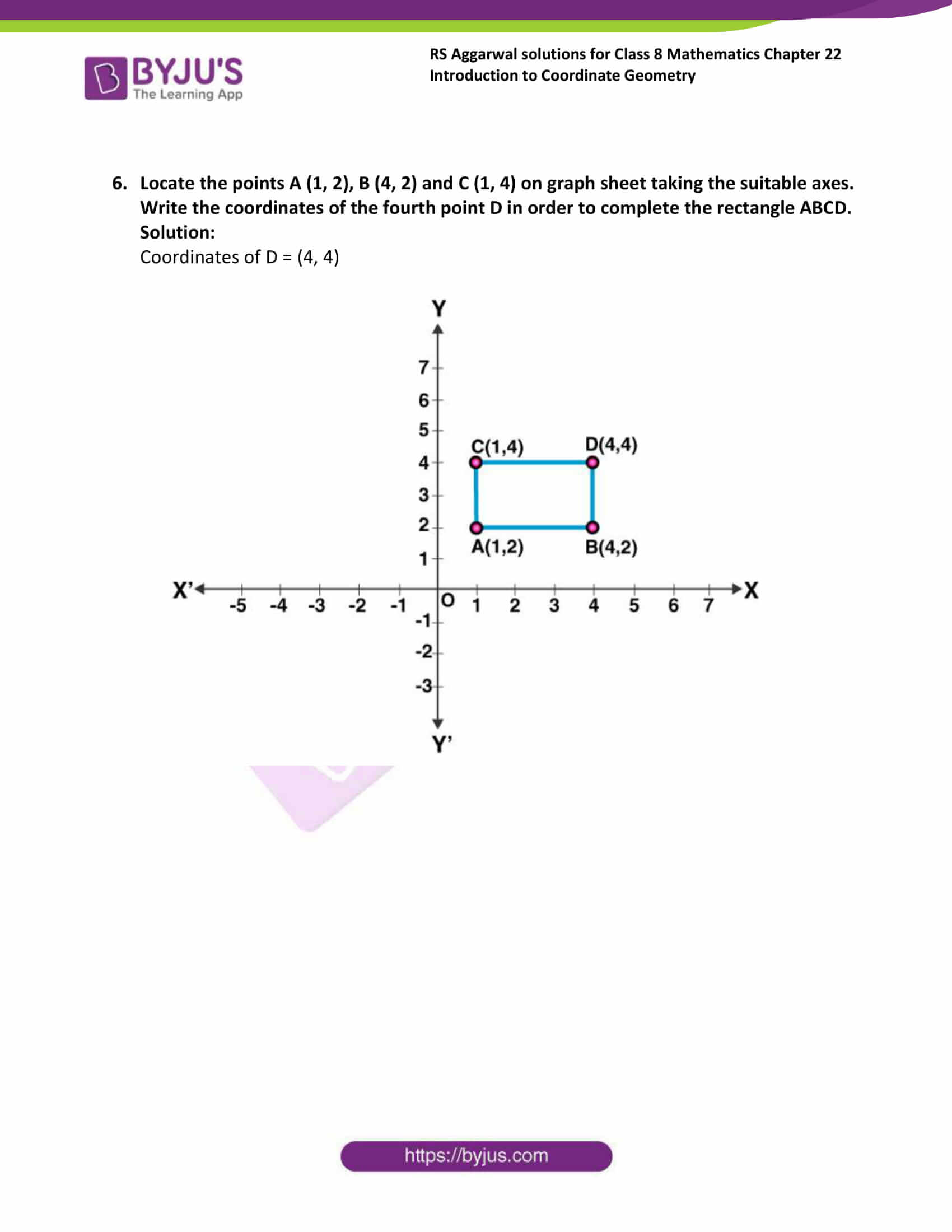### Access answers to Maths RS Aggarwal Solutions for Class 8 Chapter 22 – Introduction to Coordinate Geometry Exercise 22A

Exercise 22A Page No: 247

1. Write the abscissa of the each of the following points:

(i) (0,5)

(ii) (3,7)

(iii) (-2,4)

(iv) (6, -3)

Solution:

(i) Abscissa of (0,5) is 0

(ii) Abscissa of (3,7) is 3

(iii) Abscissa of (-2, 4) is -2

(iv) Abscissa of (6, -3) is 6

2. Write the ordinate of the each of the following points:

(i) (4,0)

(ii) (5,2)

(iii) (1,-4)

(iv) (-10,-7)

Solution:

(i) Ordinate of (4,0) is 0

(ii) Ordinate of (5,2) is 2

(iii) Ordinate of (1,-4) is -4

(iv) Ordinate of (-10,-7) is -7

3. On a graph paper, plot the each of the following points:

(i) A(4,3)

(ii) B(-2,5)

(iii) C(0,4)

(iv) D(7,0)

(v) E(-3,-5)

(vi) F(5,-3)

(vii) G(-5,-5)

(viii) H(0,0)

Solution: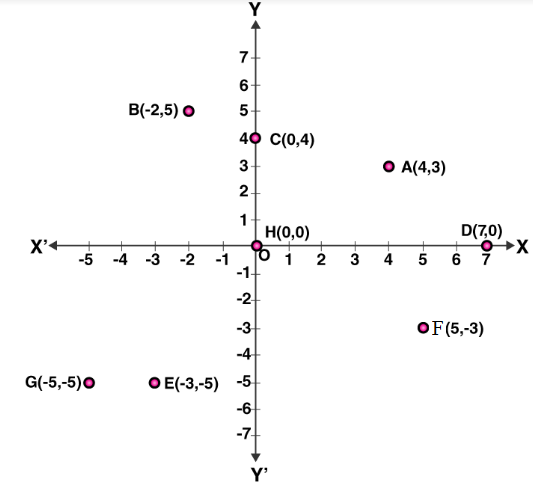4. Plot each of the following points on the graph sheet. Verify that they lie on a line.

(i) A(4,0), B(4,2), C(4,2.5) and D(4,6)

(ii) P(1,1), Q(2,2), R(3,3) and S(4,4)

(iii) L(6,2), M(5,3), N(3,5) and O(2,6)

Solution:

(i) After joining the points A (4, 0), B (4, 2), C (4, 2.5) and D (4, 6) we can see the given points forms a straight line.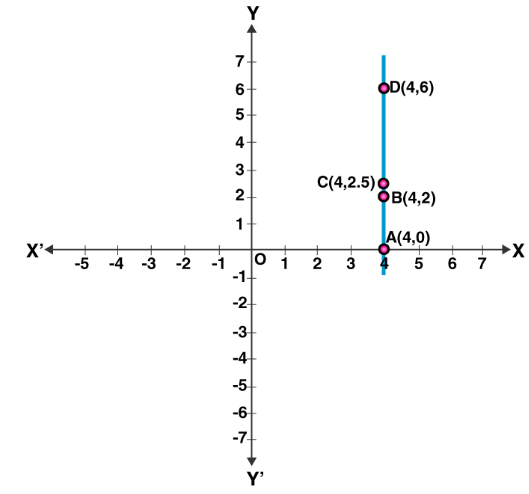(ii) After joining the points P (1, 1), Q (2, 2), R (3, 3) and S (4, 4) we can see the given points forms a straight line.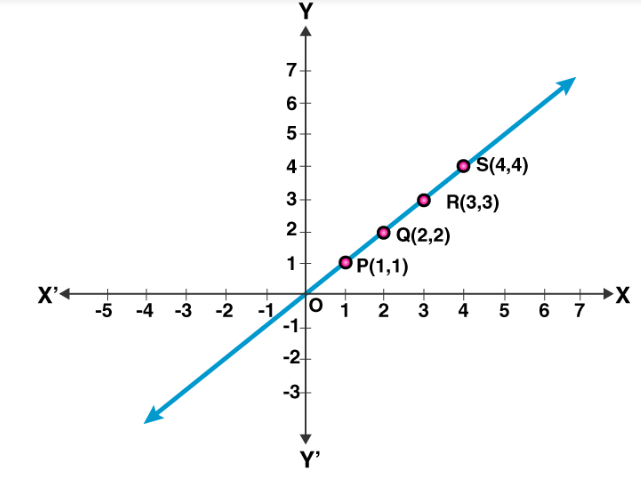(iii) After joining the points L (6, 2), M (5, 3), N (3, 5) and O (2, 6) we can see the given points forms a straight line.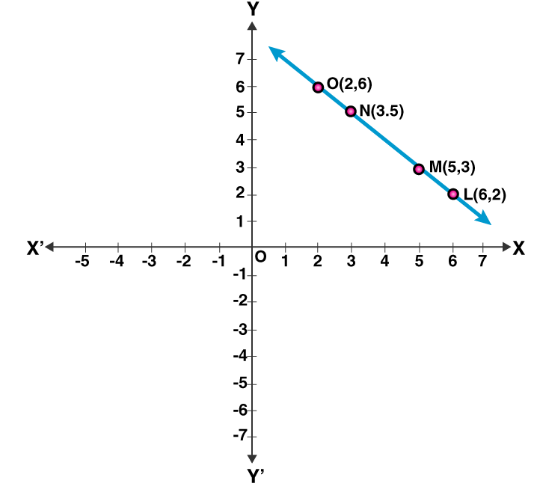5. Plot the given points on a graph sheet and check if they lie on a straight line. If not, name the shape when they joined in the order.

(i) A(0,2), B(0,3.5), C(0,5), D(0,6)

(ii) P(1,3), Q(1,5), R(3,3), S(3,5)

(iii) E(4,5), F(5,5), G(5,7),H(6,5)

(iv) L(2,0), M(2,3), N(0,3),O(0,0)

(v) J(4,3), K(6,1), L(6,5),M(4,7)

Solution:

(i) After joining the points A (0, 2), B (0, 3.5), C (0, 5), D (0, 6) we can see they form a straight line.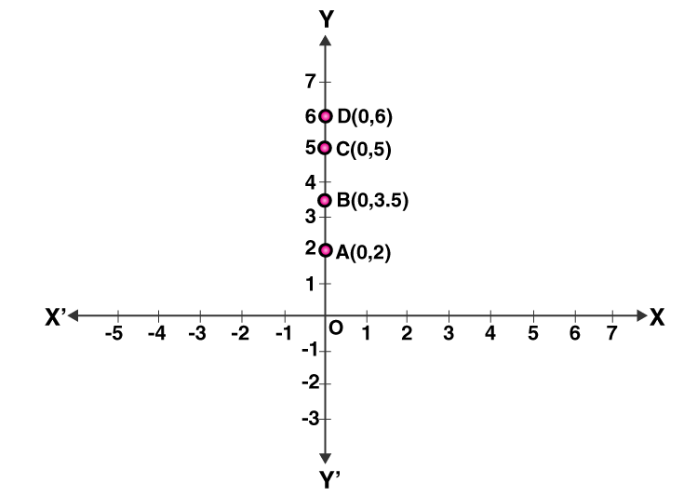(ii) After joining the points P (1, 3), Q (1, 5), R (3, 3), S (3, 5) we can see they form a square.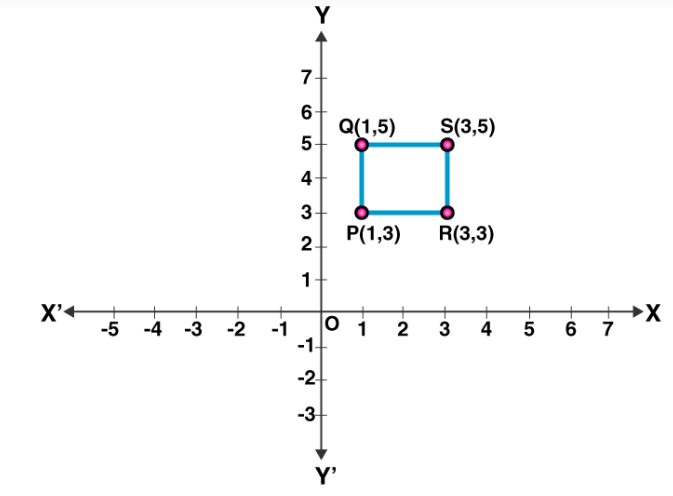(iii) After joining the points E (4, 5), F (5, 5), G (5, 7), H (6, 5) we can see they form a triangle.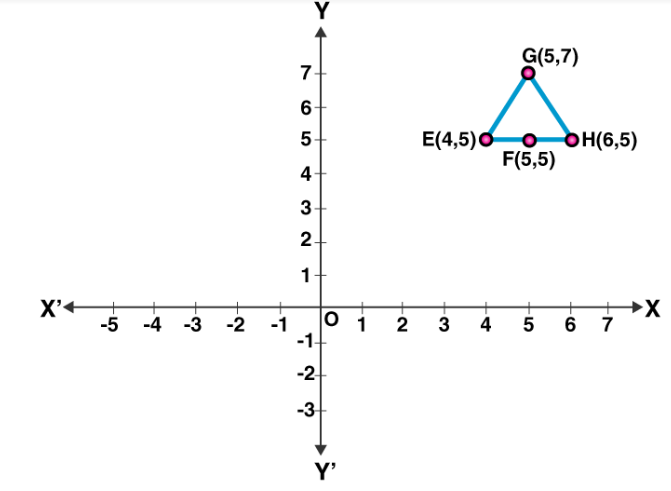(iv) After joining the points L (2, 0), M (2, 3), N (0, 3), O (0, 0) we can see they form a rectangle.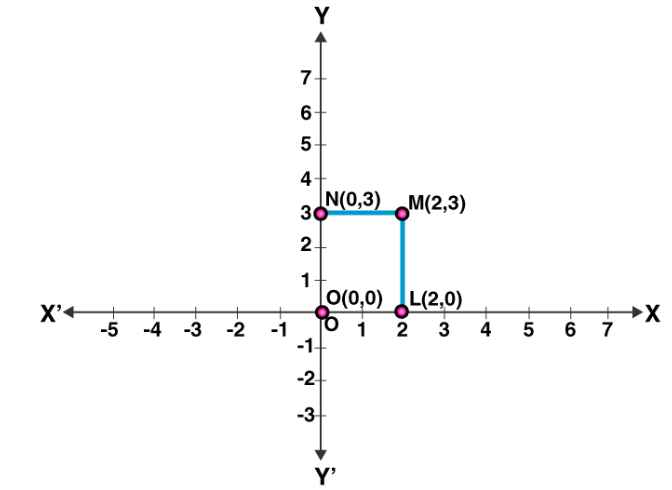(v) After joining the points J (4, 3), K (6, 1), L (6, 5), M (4, 7) we can see they form a parallelogram.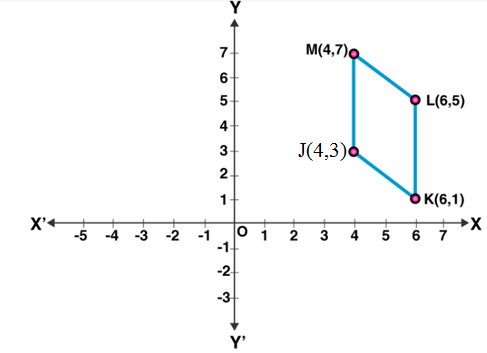6. Locate the points A (1, 2), B (4, 2) and C (1, 4) on graph sheet taking the suitable axes. Write the coordinates of the fourth point D in order to complete the rectangle ABCD.

Solution:

Coordinates of D = (4, 4)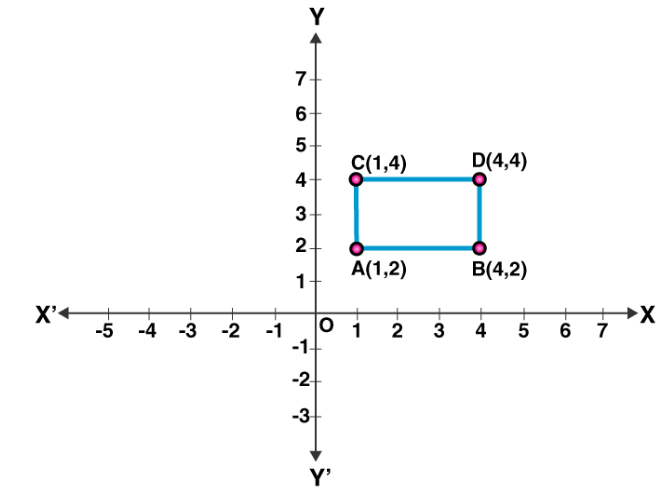### Access another exercise of RS Aggarwal Solutions for Class 8 Maths Chapter 22 – Introduction to Coordinate Geometry

Exercise 22B Solutions 7 questions

## RS Aggarwal Solutions for Class 8 Maths Chapter 22 – Introduction to Coordinate Geometry Exercise 22A

Exercise 22A of RS Aggarwal Solutions for Class 8 Maths Chapter 22 Introduction to Coordinate Geometry deals with the basic concepts related to the cartesian plane and coordinate geometry. We can say that this exercise mainly deals with the basic concepts of the cartesian plane that the students have learned in this Chapter. Some of the topics focused prior to Exercise 22A include the following.

• Coordinate axis
• Order pair
• Coordinates of a point
• Plotting the graph

The RS Aggarwal Solutions can help the students in practising and learning each and every concept as it provides solutions to all questions asked in the RS Aggarwal textbook.